Get instant live expert help with Excel or Google Sheets“My Excelchat expert helped me in less than 20 minutes, saving me what would have been 5 hours of work!”

#### Post your problem and you’ll get Expert help in seconds.

Your message must be at least 40 characters
Our professional Expert are available now. Your privacy is guaranteed.

# Excel AVERAGE Function Examples – AVERAGEIF Excel, Calculate Average Excel

Excel offers several options to find the average of a range of numbers. You could use a formula to do this, but you can also use the AVERAGE function. This is a list of the various tutorials related to using the AVERAGE function. You can use these to learn the function as well as to solve issues in Excel.

If you aren’t able to find what you need or are in a time crunch, Excelchat can help you with any Excel-related problem 24/7.Use these tutorials to learn about the AVERAGE function in Excel.

## How to Calculate the Average Growth Rate in Excel

When you are creating strategic plans or analyzing data, you’ll need to know how to calculate different rates of growth. This in-depth tutorial shows you how to calculate the average growth rate in Excel using the AVERAGE function. This includes the Average Annual Growth Rate (AAGR) and the Compound Average Growth Rate (CAGR). It not only includes examples and illustrations, but there is also a detailed video tutorial that you can watch.

## How to Calculate a Rolling Average in Excel

Another popular calculation involving the AVERAGE function is the rolling average. This might also be referred to as a moving mean, running average, or moving average. This piece describes how to do this calculation with an example. There is also a video tutorial included.

## How to Calculate the Weighted Average in Excel

When the values that you want to average have various degrees of relevance, you’ll want to produce a weighted average. This is a step-by-step guide to calculating the weighted average of a range of numbers in Excel using the SUM and SUMPRODUCT functions. There are examples and illustrations included.

## How to Calculate an Average Without Zeros or Blank Cells

What if you’re trying to produce an average from a range, but there are zeros or blank cells included? If those were included in your calculation, this could skew the result. This tutorial shows how to calculate an average that will also ignore zeros and blank cells. There is an example with illustrations as well as a video tutorial included.

## Average Last 5 Values in Columns

If you have a large dataset, you may only want the average of the most recent figures. This piece explains how to find the average of the last five values in a column, which is a figure you can adapt to your requirements. It includes examples and illustrations to help with learning.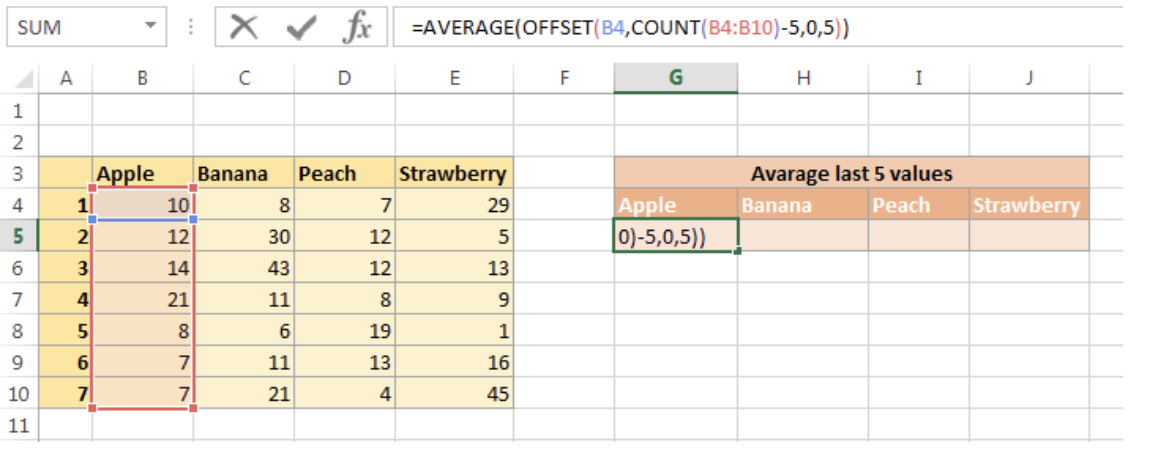Find the average of the last five values in a column.

## How to Calculate the Average Response Time Per Month in Excel

Does your Excel sheet track response times? If so, it might be helpful to create monthly figures that show the average response time per month so that you can track progress. This piece explains how to do this using the AVERAGEIFS function. It provides the generic syntax of the function and then a specific example with illustrations.

## How to Calculate the Average Top 3 Score in Excel

When you have a dataset of scores, you can use Excel to extract useful figures. This tutorial shows the reader how to pull the top three scores from a range and then how to produce the AVERAGE of those scores. The examples explain how to set up the data and then provides the formula for the operation.

## How to Calculate the Geometric Mean in Excel

The GEOMEAN function in Excel is used to produce the average rate per period for a set of figures that are compounded over multiple periods. This piece explains how to calculate this in Excel with an example set of data that includes illustrations.

## Discover How to Calculate an Exponential Moving Average in Excel

When recent data is more important than past data, you might want to opt for an exponential moving average (EMA). This tutorial explains this type of average and then acts as a step-by-step guide to produce this using the Data Analysis Add-on in Excel. The reader receives guidance on installing and using this add-on to produce an EMA.

## Here’s a Guide on Finding the Median in a Pivot Table

Pivot tables add an extra layer of complication to some calculations. This piece describes how you can find the median in a pivot table by combining the IF and MEDIAN functions. There are examples and illustrations to help with guidance.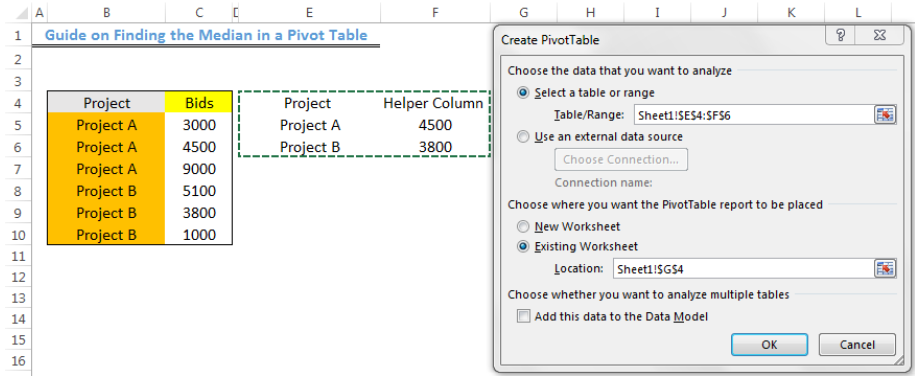Learn how to find the median in a pivot table.

## Learn How to Find a Median if Your Data Meets Certain Criteria

You might have a large dataset and only want the median of certain numbers if they meet your requirements. This tutorial shows you how to calculate the median of a range only if the figures meet your criteria. It uses the MEDIAN and IF functions. The guide shows you how to set up the data and then create the right formula for this action.

## AVERAGE and Ignore Errors

What if a calculation for an average in Excel returns an error? This might be a challenge if you have a large set of data to review. Instead of dealing with finding and solving errors, this piece shows how to create an average in Excel and also ignore any errors that might exist within the data. It includes an example and illustrations.

## How to Calculate a Weighted Average in an Excel Pivot Table

When working with pivot tables, you can also produce a weighted average of your selected data. This tutorial shows how to do this in Excel by providing some detailed examples and illustrations.

## How to Calculate AVERAGE in Excel

This is a high-level tutorial piece that deals with Excel’s AVERAGE, AVERAGEA, and AVERAGEIFS functions. It explains how each one is employed, gives the basic syntax for each, and provides examples and illustrations for their use.

## Average Number

This is another tutorial piece that deals specifically with the AVERAGE function. Anyone who doesn’t know how to create an average in Excel can follow this step-by-step guide to producing an average with the example and illustrations included here.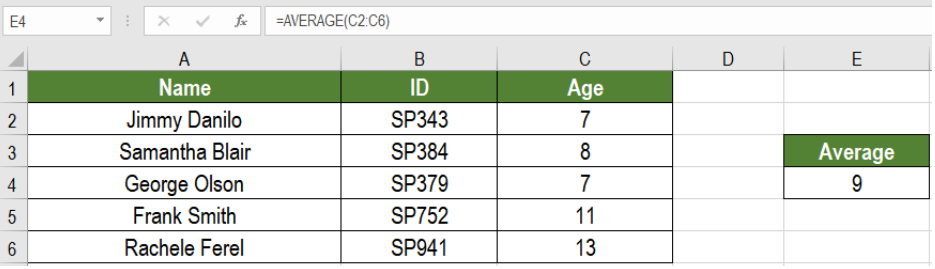Learn how to calculate an average in Excel with this guide.

## How to Use the AVERAGEIF Function in Excel

Excel’s AVERAGEIF function allows you to calculate an average when you also have some criteria. This piece explains how this function works and provides a detailed example with illustrations as a tutorial.

## Here is How You Can Calculate the Mean in Excel

Another way to think about an average is to find to the “mean” of a range of numbers. This is a guide to finding the mean of any range of numbers in Excel using the AVERAGE function. The guide explains the arguments in the formula and then provides an example of calculating the mean.

## Learn How to Calculate the Weighted Average in Excel

You can provide various weights to your figures in Excel before you calculate an average. In other words, you give more importance to some figures over others. This piece shows how to calculate the weighted average in Excel using the SUM and SUMPRODUCT functions. There are an example and illustrations as a guide.

## Discover How to Calculate the Median in Excel

The median is the middle number in a range of cells instead of the mean or average. This piece shows how to find the median of a range of numbers using the MEDIAN function. It provides the syntax for the function as well as a detailed example and illustrations.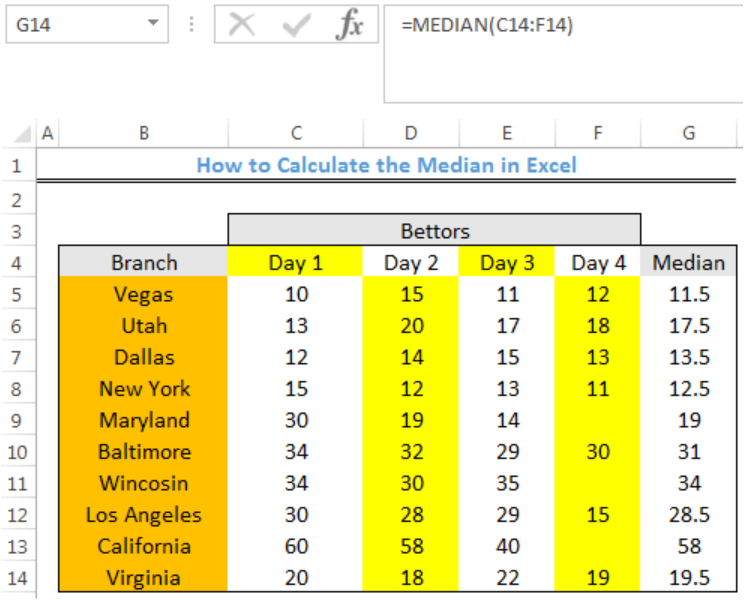Learn how to find the median of a range in Excel.

## Learn How to Use the AVERAGEIFS Function in Excel

When you have more than one criteria before calculating an average, this is when you will use the AVERAGEIFS function. This tutorial is a guide for using AVERAGEIFS with a variety of different operators such as wildcards and partial matching.

## Here’s a Guide on Finding the Moving Average in Excel

This is another tutorial that shows the reader how to find the moving average in Excel. This is useful when, for instance, you want an average for the past four weeks. It uses the AVERAGE function, and there is an example with illustrations.

## Learn How to Use the AVERAGEIF Function in Google Sheets

This is a tutorial that is specific to using Google Sheets and shows how to use the AVERAGEIF function to find the mean with there is a specified criteria. It includes an example and illustrations.

## Here is How You Can Find a Running Average in Excel

A running average is one that continuously changes as data is added. This piece explains how to produce a running average calculation in Excel using the SUM function. It includes an example.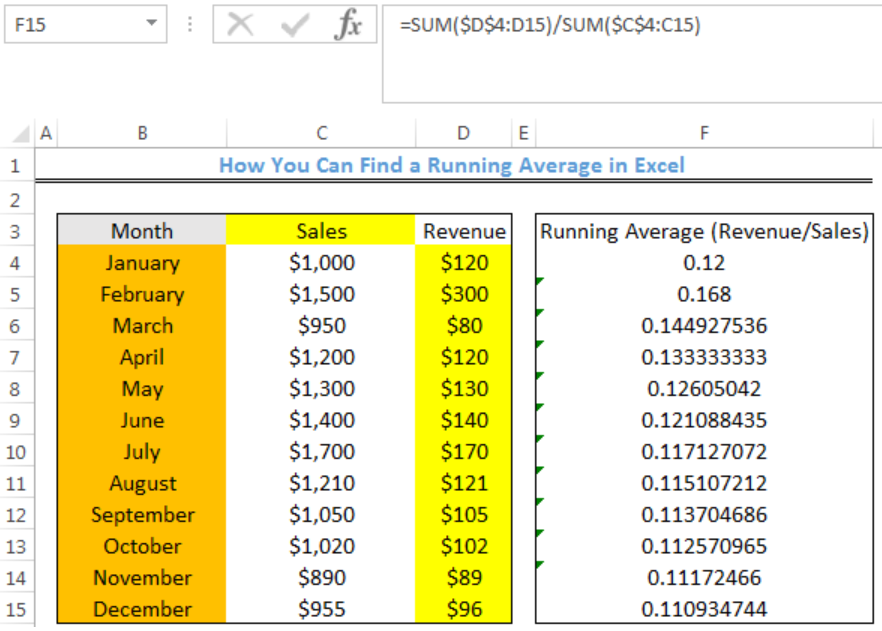Learn how to find a running average in Excel.

## Here is How to Find the Average of Data in Excel Ignoring Zeros

When you include zeros in your average calculations, it brings the numbers down. You can exclude zeros by using the AVERAGEIF function and putting in certain criteria. This tutorial and example show you how.

Hopefully, you found what you needed in this list. If you didn’t or just want some more personalized help, Excelchat is always standing by to provide assistance. We have Excel experts to deliver fast and friendly problem-specific help, whether it deals with learning Excel or resolving a problem.

Jump on a live chat session now to get started. Your first session is always free.